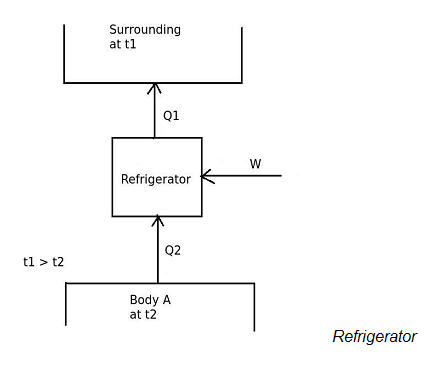# The coefficient of performance (COP)ref of refrigerator is given by.

## Considering following schematic diagram of refrigerator, the coefficient of performance (COP)ref of refrigerator is given by,a. [COP]ref = Q1/Q2
b. [COP]ref = Q2/Q1
c. [COP]ref = Q1/W
d. [COP]ref = Q2/W

Correct Answer : d. [COP]ref = Q2/W

Explanation :

Refrigerator is a device, which operates in a cycle and maintains the temperature a particular body lower than the surrounding temperature. In the above diagram of a refrigerator the body A is maintained at a temperature (t1) lower that the temperature of surrounding (t2). So the desired effect is concentrated at the body A that is Q2, not at the surrounding. The COP is calculated by the formula,
COP = Desired effect / Work input
COP = Q2 / W